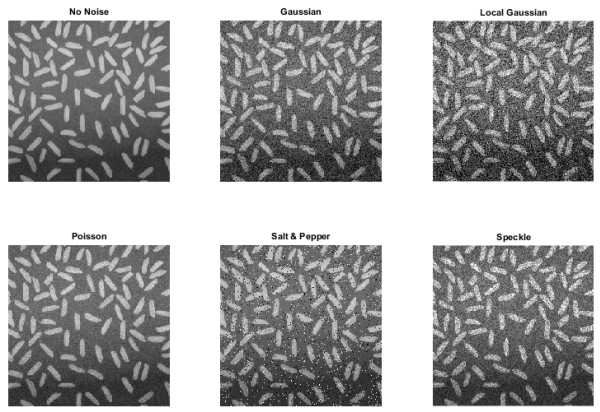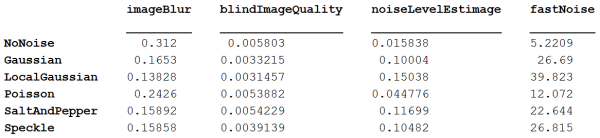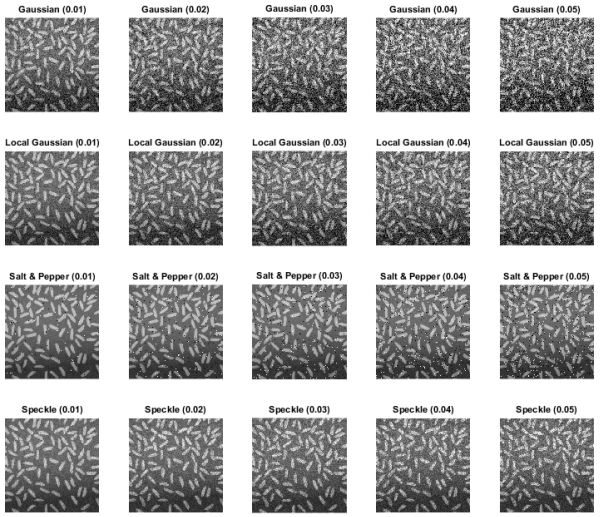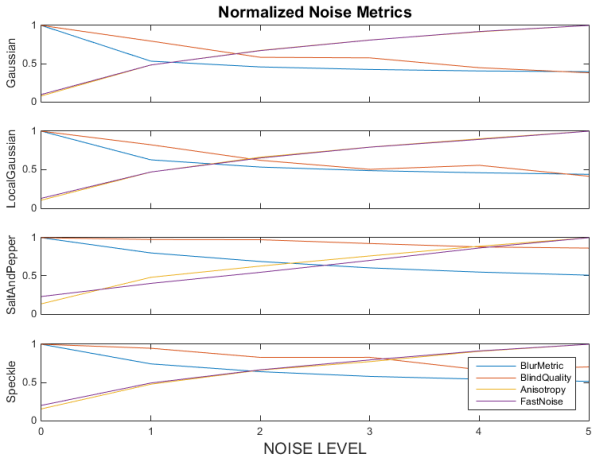# How noisy are your images…revisited

### Contents

Brett's Pick this week includes nods to two readers, and a submission called Fast Noise Estimation in Images by Tolga Birdal.

In my last post, back in September, I discussed several files for quantifying noise in images, without reference to a gold-standard image. I Picked and compared three submissions that simplified the quantification of image noise in isolated images. All of my tests, however, were conducted against image files that I had artificially blurred. Eric and Marco correctly took me to task for that, pointing out that 'blur' is not synonymous with 'noise' (though maybe we can agree that it can be a type of noise.)

Marco further pointed me to Tolga's submission, a fiendishly simple function that convolves the test image with a reference kernel to quantify noise.

In today's post, I would like to revisit noise quantification using my previous Picks, and Tolga's, and to look at their application to images containing different types of noise. I will again use the "rice.png" image that ships with the Image Processing Toolbox to consider how each does in quantifying the five types of noise-- "contant Gaussian," "locally varying Gaussian," Poisson, salt-and-pepper, and speckle--created by the imnoise function. To start, I'm going to use default input parameters (where possible) in the calls to imnoise.

#### Create Noisy Images

img = cell(6,1);
subplot(2,3,1)
imshow(img{1});title('No Noise');
img{2} = imnoise(img{1},'gaussian');
subplot(2,3,2)
imshow(img{2});title('Gaussian');
img{3} = imnoise(img{1},'localvar',0.05*rand(size(img{1})));
subplot(2,3,3)
imshow(img{3});title('Local Gaussian');
img{4} = imnoise(img{1},'poisson');
subplot(2,3,4)
imshow(img{4});title('Poisson');
img{5} = imnoise(img{1},'salt & pepper');
subplot(2,3,5)
imshow(img{5});title('Salt & Pepper');
img{6} = imnoise(img{1},'speckle');
subplot(2,3,6)
imshow(img{6});title('Speckle');#### Quantifying Noise

Now consider how each of the tools we've discussed:

quantifies noise in each of these images:

for ii = 1:6
imageBlur(ii) = blurMetric(img{ii});
blindImageQuality(ii) = blindimagequality(img{ii},2);
noiseLevel(ii) = NLEstimate(img{ii});
fastNoiseEstimate(ii) = estimate_noise(img{ii});
end

#### Tabulate Results

NoiseTypes = {'NoNoise';'Gaussian';'LocalGaussian';'Poisson';'SaltAndPepper';'Speckle'};
Results = table(imageBlur,blindImageQuality,noiseLevelEstimage,fastNoise,...
'RowNames',NoiseTypes');#### Interpretations?

Interpreting this table is difficult and subjective. We can't necessarily compare metrics (which are on different scales), and we can't assess how each metric reflects different levels of noise. So let's do another series of analyses, increasing the noise successively, and recalculating the metrics. We can then plot some trends.

#### Improving the analysis

Let's increment four types of noise (omitting Poisson noise, for which imnoise is not adjustable) five times and recompute the metrics:

noNoise = imread('rice.png');
index = 0;
for noiseType = 1:4
% Loop over noise types
for noiseLevel = 1:5
index = index + 1;
level = 0.01*noiseLevel;
% Create noisy images and loop over metrics
switch noiseType
case 1
str = ['Gaussian (' num2str(level) ')'];
img{noiseType} = imnoise(noNoise,'gaussian',0,level);
case 2
str = [' Local Gaussian (' num2str(level) ')'];
img{noiseType} = imnoise(noNoise,'localvar',level*rand(size(noNoise)));
case 3
str = ['Salt & Pepper (' num2str(level) ')'];
img{noiseType} = imnoise(noNoise,'salt & pepper',level);
case 4
str = ['Speckle (' num2str(level) ')'];
img{noiseType} = imnoise(noNoise,'speckle',level);
end
subplot(4,5,index);
imshow(img{noiseType});title(str);
imageBlur(noiseType,noiseLevel) = blurMetric(img{noiseType});
blindImageQuality(noiseType,noiseLevel) = blindimagequality(img{noiseType},2);
noiseLevelEstimage(noiseType,noiseLevel) = NLEstimate(img{noiseType});
fastNoise(noiseType,noiseLevel) = estimate_noise(img{noiseType});
end
end#### Plotting the results

f = figure;
set(f,'DefaultAxesFontsize',8)
NoiseTypes = {'Gaussian';'LocalGaussian';'SaltAndPepper';'Speckle'};
for noiseType = 1:4
ax = subplot(4,1,noiseType);
noise = [Results{1,1},imageBlur(noiseType,:)];
plot(noise/max(noise));
if noiseType == 1
title('Normalized Noise Metrics','fontsize',12);
end
hold on
noise = [Results{1,2},blindImageQuality(noiseType,:)];
plot(noise/max(noise));
noise = [Results{1,3},noiseLevelEstimage(noiseType,:)];
plot(noise/max(noise));
noise = [Results{1,4},fastNoise(noiseType,:)];
plot(noise/max(noise));
ax.XTick = 1:6;
ax.XTickLabel = [];
ylabel(NoiseTypes{noiseType})
end
ax.XTickLabel = 0:5;
xlabel('NOISE LEVEL')
legend(MetricNames)#### Conclusions

Conclusions are easier to draw now. For the most part, we can see metric values monotonically increasing or decreasing with the amount of noise. (The trend is less important than the monotonicity; we can easily think of the metric values as quantifying image quality.)

One final comment: it's very possible--maybe even likely--that these metrics can be tweaked to give better results, and that they will perform differently for different image and noise types. I encourage you to play around with the inputs using your own images; hopefully this discussion will give you a framework for evaluating the different noise metrics.

#### SWAG!

Swag, and thanks, are due to Eric, Marco, and Tolga!